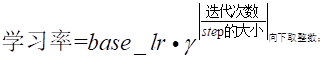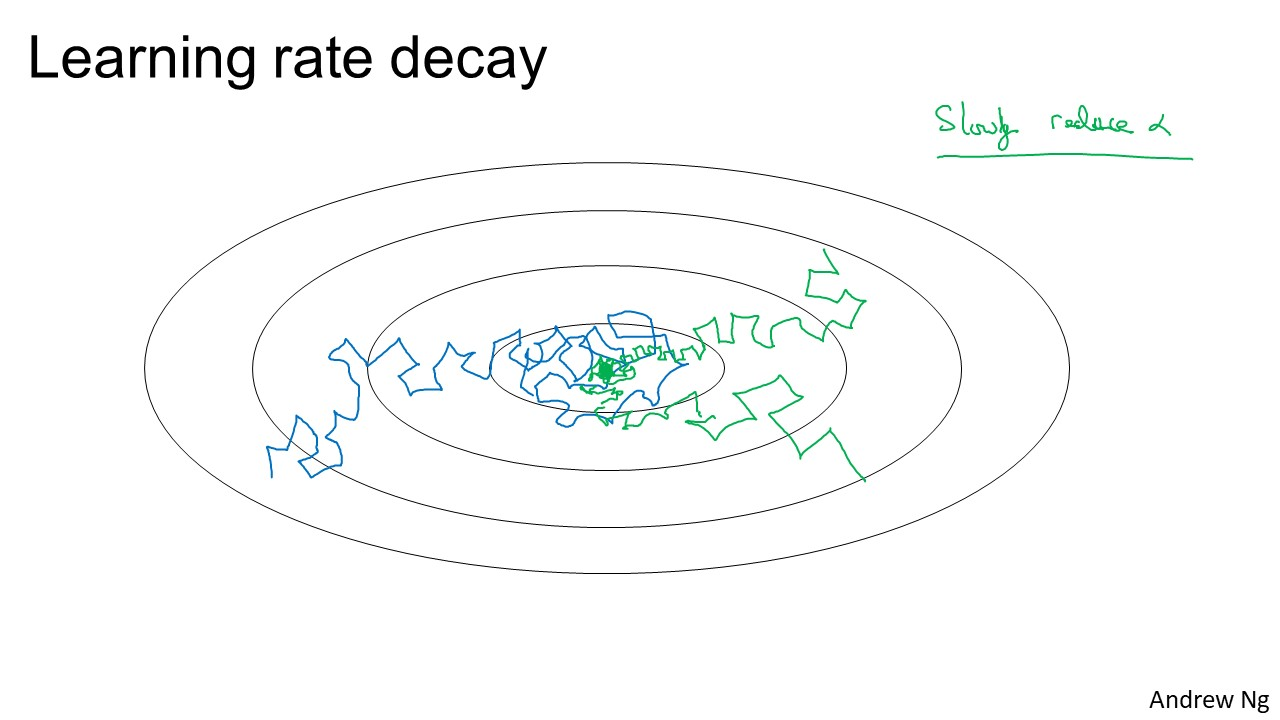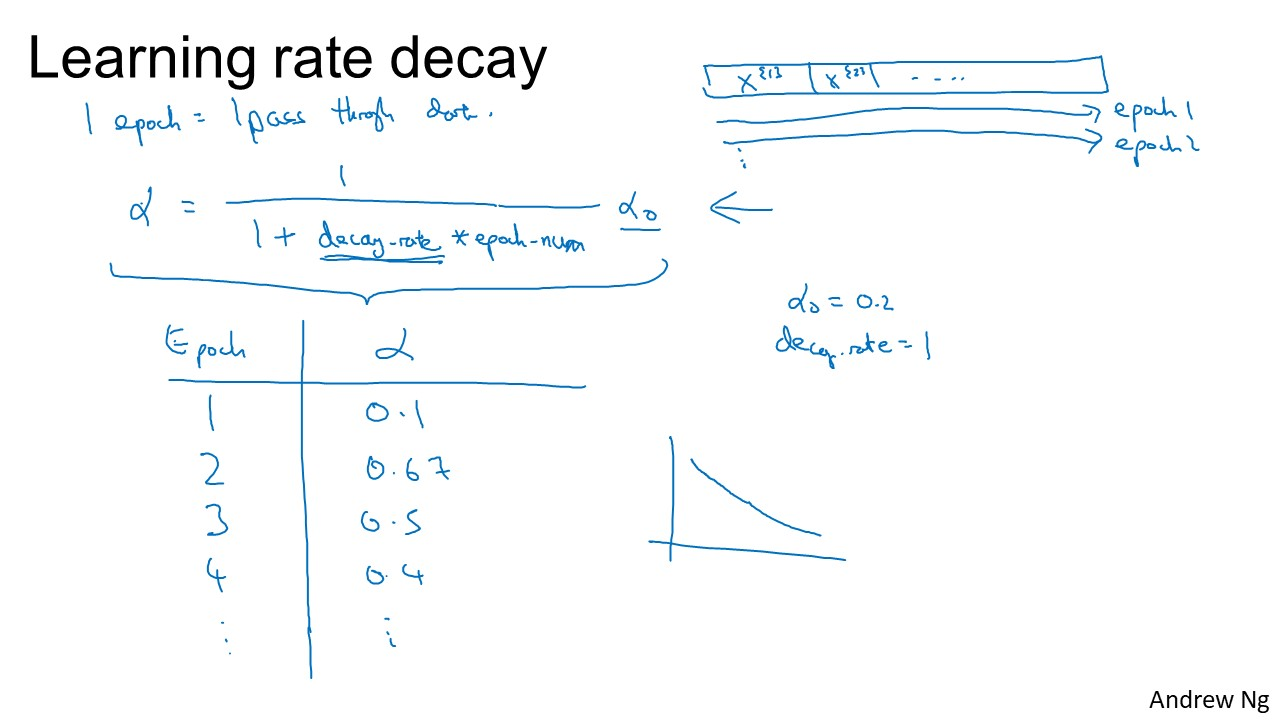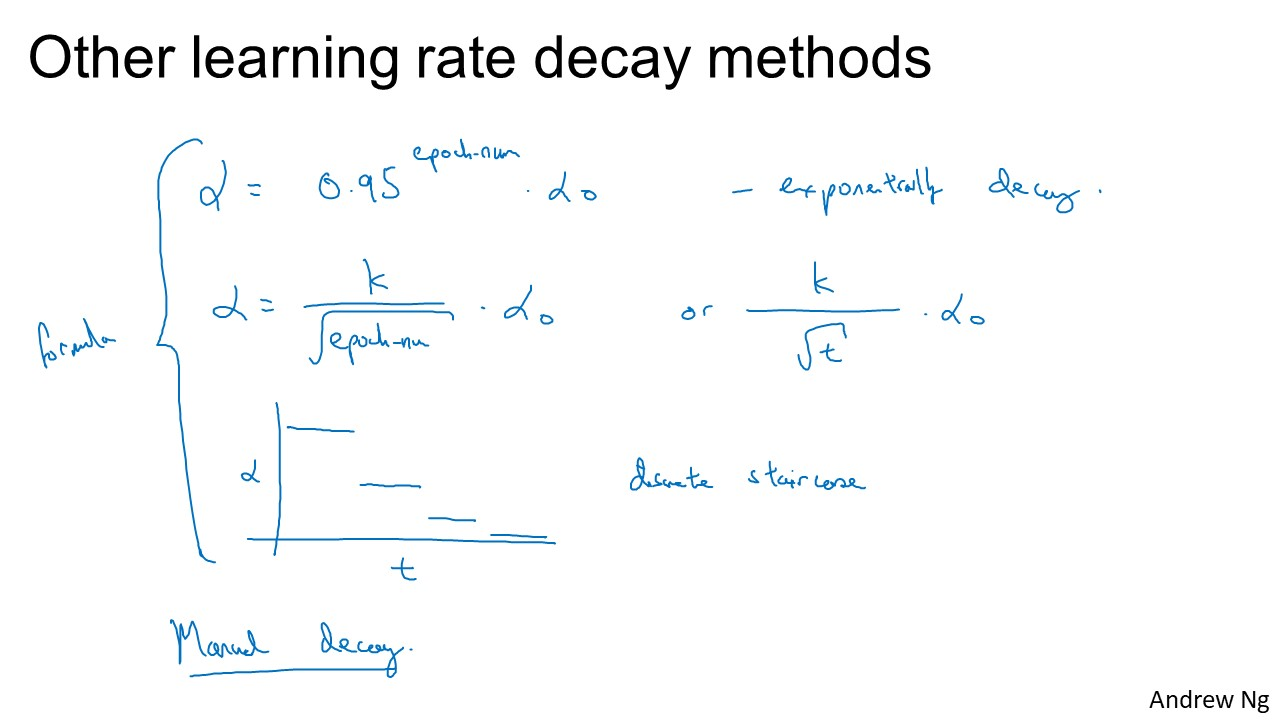• lr_scheduler = optim.lr_scheduler.CosineAnnealingLR(optimizer, T_max=5,eta_min=4e-08) lr_scheduler.step() 官网:...Exampl...
lr_scheduler = optim.lr_scheduler.CosineAnnealingLR(optimizer, T_max=5,eta_min=4e-08)
lr_scheduler.step()
官网:https://pytorch.org/docs/0.3.1/optim.html#torch.optim.lr_scheduler.CosineAnnealingLR
Example:

scheduler = optim.lr_scheduler.CosineAnnealingLR(optimizer, T_max=5,eta_min=4e-08) for epoch in range(100): scheduler.step() train(…) validate(…)

展开全文• 6）“sigmoid” - 学习率进行sigmod函数衰减，返回 base_lr ( 1/ 1＋exp ( gamma * ( iter - stepsize ) ) )，gamma设置为0.05，stepsize设置为200；     7)  inv : 其中参数  gamma  与  ...
学习率变化有以下几种常见策略：
base_lr是基础学习率，这里设置为0.1。
1）“step” - 需要设置一个stepsize参数，返回base_lr * gamma ^ ( floor ( iter / stepsize ) )，iter为当前迭代次数，gamma设置为0.4，stepsize设置100；2）“multistep”  和step相近，但是需要stepvalue参数，step是均匀等间隔变化，而multistep是根据stepvalue的值进行变化；
a.可以设置多个 stepvalue的值, 在prototxt里面也没有具体介绍， 它由参数 stepsize 与 stepvalue 决定；
b.它不是每时第刻都去计算 学习率，而是达到我们设定的stepvalue的时候，才去计算（根据方法2中的公式），然后更新学习率；
c.stepvalue 可以设置多个的

3）“fixed” - 保持base_lr不变；
4）“exp” - 返回base_lr * gamma ^ iter, iter为当前迭代次数，gamma设置为0.98；5）“poly” - 学习率进行多项式误差衰减，返回 base_lr* ( 1 - iter / max_iter ) ^ ( power )，power设置为0.9；6）“sigmoid” - 学习率进行sigmod函数衰减，返回 base_lr ( 1/ 1＋exp ( gamma * ( iter - stepsize ) ) )，gamma设置为0.05，stepsize设置为200；7)
inv : 其中参数 gamma 与 power 都需要设置；
学习率  = base_lr * (1 + gamma * iter) ^ (- power)

solver.prototxt文件是用来告诉caffe如何训练网络的。solver.prototxt的各个参数的解释如下：
base_lr 这个参数是用来表示网络的初始学习率的。这个值是一个浮点型实数。lr_policy 这个参数是用来表示学习率随着时间是如何变化的。值是字符串，需要加""。学习率变化的可选参数有： “step”——需要设置stepsize。根据gamma参数和stepsize参数来降低学习率，base_lr * gamma ^ (floor(iter / stepsize))。iter是当前迭代次数。学习率每迭代stepsize次变化一次。 “multistep”——与step类似，需要设置stepvalue，学习率根据stepvalue进行变化。 “fixed”——学习率base_lr保持不变。 “inv”——学习率变化公式为base_lr * (1 + gamma * iter) ^ (- power) “exp”——学习率变化公式为base_lr * gamma ^ iter} “poly”——学习率以多项式形式衰减，到最大迭代次数时降为0。学习率变化公式为base_lr * (1 - iter/max_iter) ^ (power)。 “sigmoid”——学习率以S型曲线形式衰减，学习率变化公式为base_lr * (1 / (1 + exp(-gamma * (iter - stepsize))))。gamma 这个参数表示学习率每次的变化程度，值为实数。stepsize 这个参数表示什么时候应该进行训练的下一过程，值为正整数。主要用在lr_policy为step的情况。stepvalue 这个参数表示什么时候应该进行训练的下一过程，值为正整数。主要用在lr_policy为multistep的情况。max_iter 这个参数表示训练神经网络迭代的最大次数，值为正整数。momentum 这个参数表示在新的计算中要保留的前面的权重数量，值为真分数，通常设为0.9。weight_decay 这个参数表示对较大权重的惩罚（正则化）因子。值为真分数。 This parameter indicates the factor of (regularization) penalization of large weights. This value is a often a real fraction.solver_mode 这个参数用来表示求解神经网络的模式——值为CPU or GPU。snapshot 这个参数用来表示每迭代多少次就应该保存snapshot的model和solverstate，值为正整数。snapshot_prefix: 这个参数用来表示保存snapshot时model和solverstate的前缀，值为带引号的字符串。net: 这个参数表示训练网络所在的位置，值为带引号的字符串。test_iter 这个参数表示 这个参数表示每个test_interval进行多少次test迭代，值为正整数。test_interval 这个参数表示什么时候进行数据的测试，值为正整数。test_initialization: true/false 是否进行第一次测试display 这个参数用来表示什么时候将输出结果打印到屏幕上，值为正整数，表示迭代次数。type 这个参数表示训练神经网络采用的反向传播算法，值为带引号的字符串。可选的值有： Stochastic Gradient Descent “SGD”——随机梯度下降，默认值。 AdaDelta “AdaDelta”——一种”鲁棒的学习率方法“，是基于梯度的优化方法。 Adaptive Gradient “AdaGrad”——自适应梯度方法。 Adam “Adam”——一种基于梯度的优化方法。 Nesterov’s Accelerated Gradient “Nesterov”——Nesterov的加速梯度法，作为凸优化中最理想的方法，其收敛速度非常快。 RMSprop “RMSProp”——一种基于梯度的优化方法。

展开全文• learning rate decay 学习速率衰减 对于mini-batch gradient descent，其梯度下降可能会围绕最优点附近徘徊，但是由于learning rate不变，可能不会进一步靠近最优点（蓝色路径） 如果learning rate会随着学习...
learning rate decay 学习速率衰减对于mini-batch gradient descent，其梯度下降可能会围绕最优点附近徘徊，但是由于learning rate不变，可能不会进一步靠近最优点（蓝色路径）如果learning rate会随着学习的深入不断衰减，那么上述情况会得到改善，最终的结果和更加靠近最优点（绿色路径）方法 一

1 epoch=1 pass through data

1

e

p

o

c

h

=

1

p

a

s

s

t

h

r

o

u

g

h

d

a

t

a

1\ epoch = 1\ pass\ through\ data

α=11+decayRate∗epochNumα0

α

=

1

1

+

d

e

c

a

y

R

a

t

e

∗

e

p

o

c

h

N

u

m

α

0

\alpha = \frac{1}{1+decayRate*epochNum}\alpha_0方法 二 (exponentially decay)

α=0.95epochNumα0

α

=

0.95

e

p

o

c

h

N

u

m

α

0

\alpha = 0.95^{epochNum}\alpha_0  方法 三

α=kepochNum−−−−−−−−−√α0

α

=

k

e

p

o

c

h

N

u

m

α

0

\alpha = \frac{k}{\sqrt{epochNum}}\alpha_0

α=kt√α0

α

=

k

t

α

0

t

t

<script type="math/tex" id="MathJax-Element-6">t</script>是mini-batch的次数。  方法 四 （discrete staircase）  方法 五 （manual decay ）
展开全文• Why should learning rate decay? &nbsp; &nbsp; &nbsp; 以目前最主流的参数优化算法Gradient Descent为例，为了让梯度下降的性能更优，我们需要将学习率设定在一个合适的范围。具体来说，学习率其实...
Why should learning rate decay?

How decay learning rate?

exponential_decay:指数衰减  decayed_learning_rate = learning_rate * decay_rate ^ (global_step / decay_steps)natural_exp_decay:自然指数衰减  decayed_learning_rate = learning_rate * exp(-decay_rate * global_step)inverse_time_decay:逆时间衰减  decayed_learning_rate = learning_rate / (1 + decay_rate * global_step / decay_step)polynomial_decay:多项式衰减  global_step = min(global_step, decay_steps)  decayed_learning_rate = (learning_rate - end_learning_rate) *(1 - global_step / decay_steps) ^ (power) + end_learning_rate
参数意义decayed_learning_rate衰减后的学习率learning_rate初始学习率decay_rate衰减率global_step当前的stepdecay_steps衰减周期
展开全文机器学习 学习率 算法
• 权重衰减（weight decay）L2正则化的目的就是为了让权重衰减到更小的值，在一定程度上减少模型过拟合的问题，所以权重衰减也叫L2正则化。1.1 L2正则化与权重衰减系数L2正则化就是在代价函数后面再加上一个正则化项...神经网络 深度学习 机器学习
• 权重衰减（weight decay）与学习率衰减learning rate decay）
• 看到一篇好文章, 系统地介绍了tensorflow中的几个学习率衰减函数, 并画图展示了它们的区别: TensorFlow学习－－学习率衰减/learning rate decay
• 学习率衰减(learning rate decay) 为了防止学习率过大，在收敛到全局最优点的时候会来回摆荡，所以要让学习率随着训练轮数不断按指数级下降，收敛梯度下降的学习步长。 学习率衰减可以用以下代码实现 decayed_...深度学习 人工智能 TensorFlow
• 一、学习率衰减的概念和必要性学习率过大，在算法优化的前期会加速学习，使得模型更容易接近局部或全局最优解。但是在后期会有较大波动，甚至出现损失函数的值围绕最小值徘徊，波动很大，始终难以达到最优，如下图...深度学习
• 很多时候我们要对学习率（learning rate）进行衰减，下面的代码示范了如何每30个epoch按10%的速率衰减： def adjust_learning_rate(optimizer, epoch): &quot;&quot;&quot;Sets the learning rate to...pytorch optimizer
• 学习率衰减learning rate decay） 在训练神经网络时，使用学习率控制参数的更新速度．学习率较小时，会大大降低参数的更新速度；学习率较大时，会使搜索过程中发生震荡，导致参数在极优值附近徘徊． 为此，在...tensorflow
• 很多时候我们要对学习率（learning rate）进行衰减，下面的代码示范了如何每30个epoch按10%的速率衰减： def adjust_learning_rate(optimizer, epoch): """Sets the learning rate to the initial ...
• 在实验过程中我们可能都对learning rate的选取而苦脑过 learning rate过小：loss降低过慢 learning rate过大：loss可能达不到最优，而在最优返回震动 其比较如下图所示 解决办法 1. 使用ReducLROnPlateau 1.1 ...深度学习 Pytorch
• 很多时候我们要对学习率（learning rate）进行衰减，下面的代码示范了如何每30个epoch按10%的速率衰减： def adjust_learning_rate(optimizer, epoch): """Sets the learning rate to the initial LR decayed by 10...
• pytorch learning rate decay本文主要是介绍在pytorch中如何使用learning rate decay. 先上代码:def adjust_learning_rate(optimizer, epoch): """ 每50个epoch,权重以0.99的速率衰减 """ if epoch // 50 == 0:...
• decay-rate衰减率 epoch-num：代数 ：初始学习率 方法2： 指数衰减： 方法3： 方法4： 方法4： 手动衰减。如果一次只训练一个模型，如果花上数小时或数天来训练，看看自己的模型......